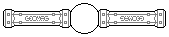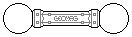Web textodigital.com
The regular tetrahedron
Perhaps because it is the simplest among the platonic solids, the regular tetrahedron seems to have played a significant role in the original Geomag design. Four balls exactly fit in the inner space of a scale-1 tetrahedron (left-hand picture), which implies very precise geometric relations among the shapes and dimensions of the Geomag pieces. It is hard to believe that this happened by chance.
The inner four balls could be regarded as another regular tetrahedron, made with zero-length rods, that is, with no rods at all. So, this zero-rod tetrahedron fits inside a single rod one. The very same geometric relations ensure that the single rod tetrahedron will fit into a double rod one, which in turn will fit into a triple rod one, an so on (right-hand picture).Regular tetrahedron filled with balls 14 pieces: 8 balls, 6 rods (97.00 g)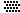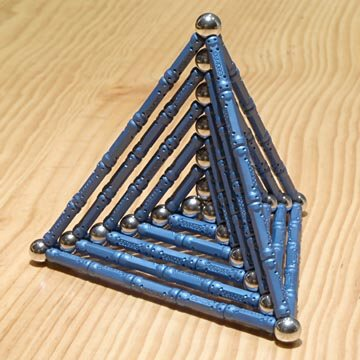Six embedded tetrahedra 114 pieces: 24 balls, 90 rods (629.40 g)The midpoints of the regular tetrahedron edges are the vertices of a regular octahedron, made of green rods in the left-hand picture. If we superimpose two tetrahedra in such a way that their edges are perpendicular and cut at midpoints (yellow and red in the right-hand picture), the intersection is that very same octahedron.
In other words, if we add four single-scale regular tetrahedra on alternate faces of a regular octahedron, we obtain a double-scale regular tetrahedron (again, left-hand picture). If we add a tetrahedron on every face of the octahedron, we obtain the body shown in the right-hand picture, equivalent to two intersecting double-scale tetrahedra, and called stella octangula.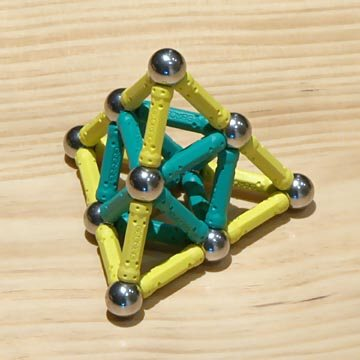Regular tetrahedron to double scale 34 pieces: 10 balls, 24 rods (198.80 g)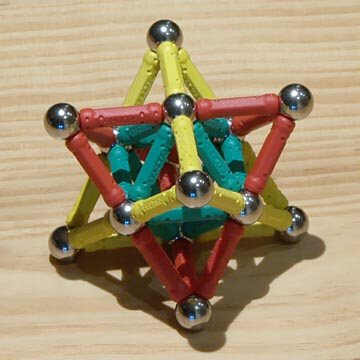Stella octangula 50 pieces: 14 balls, 36 rods (289.60 g)We could also regard the double-scale tetrahedron as built by joining four single-scale ones by their vertices. The inner octahedron turns out to be, simply, the empty space which remains in between. Similarly, to build a quadruple scale tetrahedron, we can join four double-scale ones; the empty space is now a double-scale octahedron (see left-hand picture).
Adding another four double-scale tetrahedra on the hollow faces of this octahedron, we get the double-scale stella octangula shown in the right-hand picture, in which the inner octahedron is also hollow.Tetrahedron to quadruple scale 130 pieces: 34 balls, 96 rods (743.60 g)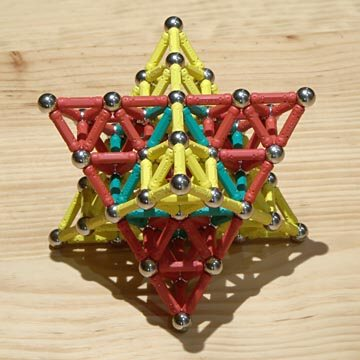Stella octangula to double scale 218 pieces: 50 balls, 168 rods (1.22 kg)The funny thing is that it seems there is no theoretical limit to this method of scaling up the tetrahedron, although the proportion of hollow space grows in each iteration. Notice that we will build the octuple-scale tetrahedron using the quadruple-scale ones just shown. Besides a hollow quadruple-scale inner octahedron, this body will contain the four hollow double-scale octahedra belonging in the component tetrahedra. And so on.
It is possible, however, for the practical limit to be at something like the fourth iteration, that is, at the regular tetrahedron to scale 16, as the one shown in the following pictures: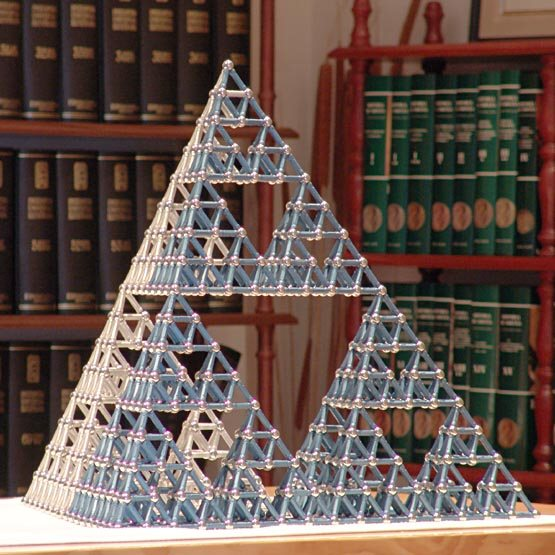Tetrahedron to scale 16 (Sierpinski, fourth iteration), side view 2050 pieces: 514 balls, 1536 rods (11.64 kg)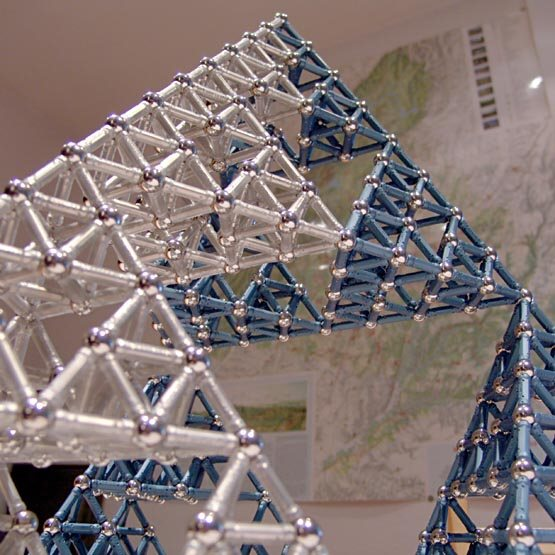Tetrahedron to scale 16 (Sierpinski, fourth iteration), bottom oblique view
Bodies so built are called Sierpinski tetrahedra or tetrix, as they are similar to the first iterations of a so-called ideal recursive geometrical object. The next iteration would be made of 6144 rods, and would have a hollow span measuring 16 rods, just like the size of the base of the previous body.
The nth iteration would have 6 × 4n rods and 2 × 4n + 2 balls.
Building objects with big planar bases, like this one, requires a truly planar table. When I built it, I was searching for a defective or misplaced piece until I realized that the construction didn't perfectly fit on my table at a certain position, while it did at others.